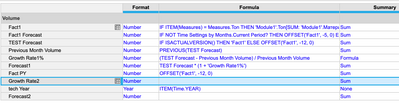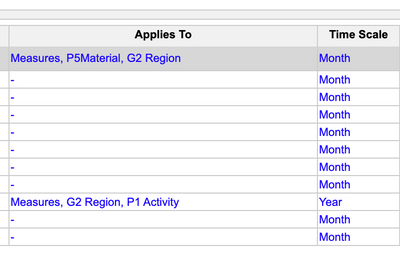# Formula for subsidiary view itemHi!

I have a question about formula in subsidiary view.

I have a line item with different dimensions.

What should I refer to in the formula so it would work (and can be seen) in subsidiary view item?

Other items in the module are in regular dimensions.. And I would like to use them in the formula for subsidiary view item.

(Regular dimensions are: Measures, P5Material, G2 Region),

And Line item is in Measures, G2Region, P1).

Thank you.

Joanna.

Tagged:

•You will need to create a mapping that bridges the difference in dimensions in the same way you would between two modules.

The module has; Measures, P5Material, G2 Region

The line item has; Measures, P1, G2Region

However, assuming that P5Material and P1 are part of the same hierarchy and P1 is a higher level then the line item will simply take the value from the module which relates to the P1 layer.

If they are not part of the same hierarchy then you will need to build a mapping table.

If multiple P5 items map into a P1 item then create a mapping table dimensioned by P5 and add a line item formatted as a the list P1. Then use SUM.

If its a 1 to 1 mapping then you can create a mapping table dimensioned by P1 and a line item formatted as P5. Then use LOOKUP.

•Hi @JoannaAn!

What formula do you want to write?
And what will be the source and what will be the receiver?
If you are going from P1 to P5, then you need LOOKUP. And if from P5 to P1, then the system will be able to add the data in the hierarchy itself (if your list if composite).

•I need to calculate Growth Rate, but within those particular dimensions. So subsidiary view applied on the Growth rate Line item.

I have a sales Volume by month in the same module. But they are in regular dimensions.

•Also other Items are calculated within regular dimensions...so they are also contains formulas...

Does it makes everything more difficult?

•What if I have other line items (which are also calculated?) does it makes everything more difficult?

I actually trying to calculate the same Growth Rate (which I did yesterday - Month-to-Month growth rate  https://community.anaplan.com/t5/Anaplan-Platform/How-to-calculate-Month-to-month-Growth-Rate/m-p/107881#M25853 ) but within changed dimensions...so it would pick only numbers within new dimensions. And yes, P5 is a part of the hierarchy with P1.

•It's hard to say, I need a screenshot of the blueprint / module.
It is likely that you can calculate the percentage of the increase in detailed measurements and put the summary =  formula. Then subsidiary view will not be needed.

•So, before I did calculations within module dimensions.

And now I need within some changed ones.

•Can you show me "Applies to"?
Based on the list of analysts and formulas you have written, you can get the correct numbers in the first line item for calculating growth. And for displaying on dashboards, use filters.

•Here is applies to

•Are P1 and P5 related hierarchies, composite directories?

First, try opening your module and pivoting so that there are years, P1 level and other dimensions. If you have a sufficient calendar, then the line item for growth may already have the correct values. You just need to set up the correct view.

If P1 and P5 are unrelated directories, then it makes sense to create a separate module and repeat the calculation.

•Yes, P5 and P1 are the same hierarchy.

I was thinking that filtering and pivoting might work.. but I was said that it's not really.

(One of my source's module is flat..that's why..But in L1 and L2 there was no anything similar..)